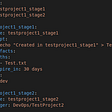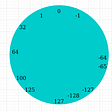# How integers are stored in memory using two’s complement

Computer machines are composed by digital memories that store information in bits (binary digit). It means that a bit is an unit — the smallest one — used to quantify computer data . The bit represents a logical value, which corresponds to a two-state device. These states are often represented as 0 or 1, and could be interpreted as “on/off”, “true/false”, etc.

On the other hand, a bit is a digit of the binary numeral system. Through this numeral system, and the combination of bits, any integer belonging to the decimal numeral system can be represented. As digital information are stored in bits, computers use binary numeral system to represent all numbers — integers, octals, hexadecimals. A byte is commonly known as a group of 8 bits.

In programming languages, like C language, it is possible to declare variables in order to store numeric data in the computer memory. The number of bits that represent integers (int) data type can change according to the computer architecture, or processing, and the compiler. In a 16-bit machine, the size of an integer is of 2 bytes, but in a 32-bit or 64-bit machine, the size is of 4 bytes. The values range of integers are shown in the following table :

Supposing there is a C program — compiled in 64-bits — where will be declared an integer variable with a value of 25 as shown below:

OUTPUT: The value of number is: 25

This integer is stored as a binary number by the computer memory:

This way it works with an unsigned and a short integer, although the last mentioned uses 16 bits. However, there are some differences when a negative value is assigned to an integer variables.

## Negative Integers

A binary with a static size represents an integer within a value range determined. For unsigned binary numbers, this value range goes from 0 to the value obtained when all digits are equally to “1”. With 2 and 4 bytes, the maximum integer value obtained by a binary of 2 and 4 bytes are shown below:

It means that their value range are determined by the following mathematical notation:

This is the way computer memory interprets unsigned integers. But for signed integers, in which a dash (“-”) is written before number when it is negative, it changes. There are some methods to represent negative numbers from a binary.

One of those methods is the 2’s complement, but to understand the 2’s complement, I will first talk about the 1’s complement which is the basis to be able to understand everything correctly :) .

## One’s complement

It is a method that represents a negative number through of inverting the values of bits of the number magnitude. Since the value first bit (also called sign bit) of the positive number is 0, when the inversion is done, this first bit is equal to 1 to indicate a negative number. The sign binary number obtained from the inversion is called complement.

The following image is an example of the process of inversion with one’s complement:

The value range of integers obtained from one’s complement is the following:

In comparison with the value range obtained with a signed binary number and its MSB, this method allows one more possible value. However, through this method a negative zero is still obtained:

## Two’s complement

It is a method to represent negative integers, similar to one’s complement. It also inverts the magnitude of the negative number but, then, it is added one (+ 1) to the complement number . It has a sign bit that allows to indicate if a number is positive or negative.

This method allows addition and subtraction with positive and negative numbers. Compared with one’s complement, the subtraction with two’s complement of two numbers , which has same magnitude value, is equal to zero.

The value range of integers obtained from two’s complement is the same as the obtained from one’s complement, shown in the Fig 9. With this method, it is not obtained a negative zero (-0), since it is added one to the zero-inverted number:

For the reasons explained above, this method is used by computer memory to store signed integers.

Supposing there is a C program that tries to print negative numbers with an integer variable (called inum) and an unsinged integer variable (called unum), like the following:

Output:
The value of inum is: -25
The value of unum is: 4294967271

It is known that unsigned integer variables only store positive numbers, but when a negative number is casted to this data type, the result is a positive number, even higher than its magnitude value — in this case, 25.

In this case, the process made by the computer consisted in determining the signed binary number of the negative integer through two’s complement method. Then, when the number was casted to an unsigned integer, the binary number was assumed as an unsigned binary number. As shown in Fig 13, the signed number binary of -25 is the same than the unsigned binary number of 4294967271.

To be successful, your desire to achieve it must be greater than your fear of failure — Bill Cosby

I hope you have enjoyed reading this little blog and have understood enough to be able to explain to someone else about how an integer is stored in memory and how the two’s complement works. See you later :) .

--

--

--

## More from Smith Flores

Love podcasts or audiobooks? Learn on the go with our new app.

## Gitlab CI — Sharing of Artifacts when one pipeline calls another## The 3 Basic Elements of Programming## You have to stop defending your favourite technology at any cost. It helps no one.## Most Important Skills When Learning to Code## map and flatMap in Scala for collections## I cried after completing my nanodegree and it was worth it## Meaningful Names (Clean Code) — Why it is important for software developers?## Mining profit withdrawal## Numbering System Edge Cases of Java## FAQ Series_101 — Form Recognizer## the differences between static and dynamic libraries